Problem title
Difficulty
Avg time to solve

Jump Game
Moderate
15 mins
Min Cost To Buy N Items
Easy
10 mins
Diamond of Stars
Easy
10 mins
Serialize and Deserialize Binary Tree
Hard
15 mins
Generate all parenthesis
Moderate
30 mins
Convert BST to Min Heap
Moderate
25 mins
Min Steps to one
Easy
15 mins
Construct BST from Level Order
Easy
15 mins
Minimum number of swaps required to sort an array
Easy
10 mins
Sort an array in wave form
Easy
10 mins15

# Convert A Given Binary Tree To Doubly Linked List

Difficulty: MEDIUM
Avg. time to solve
15 min
Success Rate
80%

Problem Statement

#### For the given binary tree :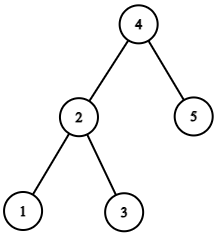``````You need to return the head to the doubly linked list.
The doubly linked list would be: 1 2 3 4 5 and can be represented as:
``````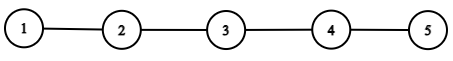##### Input Format :
``````The first line contains an Integer 'T' which denotes the number of test cases or queries to be run. Then the test cases follow.

The first line of input contains the elements of the tree in the level order form separated by a single space.
If any node does not have a left or right child, take -1 in its place. Refer to the example below.

Example :

Elements are in the level order form. The input consists of values of nodes separated by a single space in a single line. In case a node is null, we take -1 in its place.

For example, the input for the tree depicted in the below image would be :
``````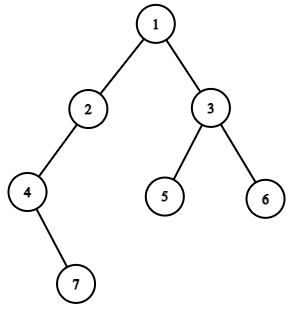``````1
2 3
4 -1 5 6
-1 7 -1 -1 -1 -1
-1 -1

Explanation  :
Level 1 :
The root node of the tree is 1

Level 2 :
Left child of 1 = 2
Right child of 1 = 3

Level 3 :
Left child of 2 = 4
Right child of 2 = null (-1)
Left child of 3 = 5
Right child of 3 = 6

Level 4 :
Left child of 4 = null (-1)
Right child of 4 = 7
Left child of 5 = null (-1)
Right child of 5 = null (-1)
Left child of 6 = null (-1)
Right child of 6 = null (-1)

Level 5 :
Left child of 7 = null (-1)
Right child of 7 = null (-1)

The first not-null node (of the previous level) is treated as the parent of the first two nodes of the current level. The second not-null node (of the previous level) is treated as the parent node for the next two nodes of the current level and so on.

The input ends when all nodes at the last level are null (-1).
``````
##### Note :
``````The above format was just to provide clarity on how the input is formed for a given tree.

The sequence will be put together in a single line separated by a single space. Hence, for the above-depicted tree, the input will be given as:

1 2 3 4 -1 5 6 -1 7 -1 -1 -1 -1 -1 -1
``````
##### Output Format :
``````For each test case, you need to return the head of the Doubly Linked List.

The output of each test case should be printed in a separate line.
``````
##### Constraints :
``````1 <= T <= 100
0 <= N <= 3000
-10 ^ 6 <= data <= 10 ^ 6 and data != -1

where 'N' is the number of nodes in the tree, 'T' represents the number of test cases and "data" denotes data contained in the node of the binary tree.

Duplicate elements can be in the right subtree or left subtree.

Time Limit: 1 sec.
``````

#### Note :

``````You are not required to print the expected output, and it has already been taken care of. Just implement the function.
``````
##### Sample Input 1 :
``````2
3 1 5 -1 2 -1 -1 -1 -1
9 6 10 4 7 -1 11 -1 -1 -1 -1 -1 -1
``````
##### Sample Output 1 :
``````1 2 3 5
4 6 7 9 10 11
``````
##### Explanation of Input 1 :
``````Here we have 2 test cases; hence there are 2 binary trees.

Test Case 1 :
``````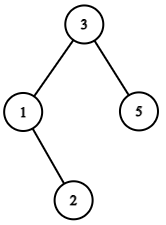``````We can see that the inorder traversal of the given tree is: 1 2 3 5.

Test Case 2 :
``````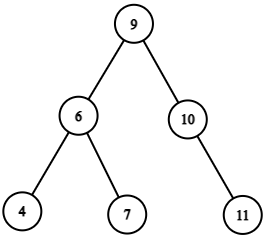``````We can see that the inorder traversal of the given tree is: 4 6 7 9 10 11.
``````
##### Sample Input 2 :
``````2
4 6 -1 5 -2 -1 -1 -1 -1
1 2 3 4 4 -1 4 -1 -1 -1 -1 -1 -1
``````
##### Sample Output 2 :
``````5 6 -2 4
4 2 4 1 3 4
``````Console